# Volumes

## Calculus 2 / Bc### 4822 Practice Problems

03:58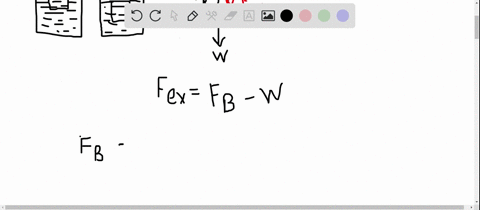Calculus for Scientists and Engineers: Early Transcendental

Archimedes' principle says that the buoyant force exerted on an object that is (partially or totally) submerged in water is equal to the weight of the water displaced by the object (see figure). Let $\rho_{w}=1 \mathrm{g} / \mathrm{cm}^{3}=1000 \mathrm{kg} / \mathrm{m}^{3}$ be the density of water and let $\rho$ be the density of an object in water. Let $f=\rho / \rho_{w} .$ If $0<f \leq 1,$ then the object floats with a fraction $f$ of its volume submerged; if $f>1,$ then the object sinks.
Consider a cubical box with sides 2 m long floating in water with one-half of its volume submerged ( $\rho=\rho_{w} / 2$ ). Find the force required to fully submerge the box (so its top surface is at the water level). (See the Guided Project Buoyancy and Archimedes' Principle for further explorations of buoyancy problems.)

Applications of Integration
Physical Applications04:45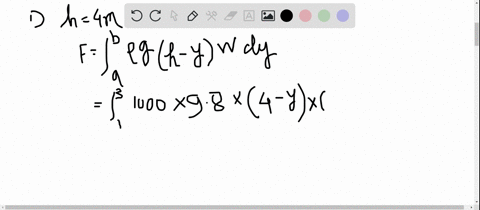Calculus for Scientists and Engineers: Early Transcendental

A large tank has a plastic window on one wall that is designed to withstand a force of $90,000 \mathrm{N}$. The square window is $2 \mathrm{m}$ on a side, and its lower edge is $1 \mathrm{m}$ from the bottom of the tank.
a. If the tank is filled to a depth of $4 \mathrm{m}$, will the window withstand the resulting force?
b. What is the maximum depth to which the tank can be filled without the window failing?

Applications of Integration
Physical Applications03:02Calculus for Scientists and Engineers: Early Transcendental

Suppose a cylindrical glass with a diameter of $\frac{1}{12} \mathrm{m}$ and a height of $\frac{1}{10} \mathrm{m}$ is filled to the brim with a $400-$ Cal milkshake. If you have a straw that is $1.1 \mathrm{m}$ long (so the top of the straw is 1 m above the top of the glass), do you burn off all the calories in the milkshake in drinking it? Assume that the density of the milkshake is $1 \mathrm{g} / \mathrm{cm}^{3}(1 \mathrm{Cal}=4184 \mathrm{J}).$

Applications of Integration
Physical Applications07:37Calculus for Scientists and Engineers: Early Transcendental

A square plate $1 \mathrm{m}$ on a side is placed on a vertical wall $1 \mathrm{m}$ below the surface of a pool filled with water. On which plate in the figure is the force greater? Try to anticipate the answer and then compute the force on each plate.

Applications of Integration
Physical Applications08:23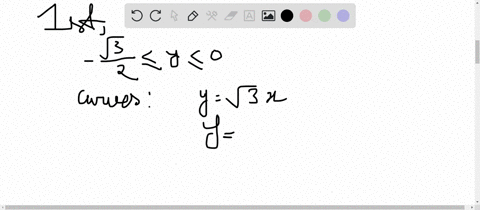Calculus for Scientists and Engineers: Early Transcendental

A plate shaped like an equilateral triangle $1 \mathrm{m}$ on a side is placed on a vertical wall $1 \mathrm{m}$ below the surface of a pool filled with water. On which plate in the figure is the force greater? Try to anticipate the answer and then compute the force on each plate.

Applications of Integration
Physical Applications04:49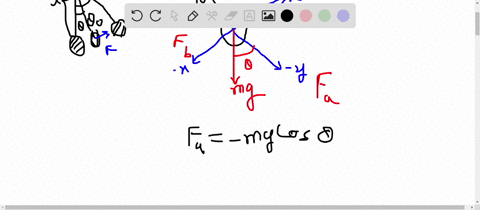Calculus for Scientists and Engineers: Early Transcendental

A body of mass $m$ is suspended by a rod of length $L$ that pivots without friction (see figure). The mass is slowly lifted along a circular arc to a height $h$
a. Assuming that the only force acting on the mass is the gravitational force, show that the component of this force acting along the arc of motion is $F=m g \sin \theta.$
b. Noting that an element of length along the path of the pendulum is $d s=L d \theta,$ evaluate an integral in $\theta$ to show that the work done in lifting the mass to a height $h$ is mgh.
FIGURE CAN'T COPY

Applications of Integration
Physical Applications03:03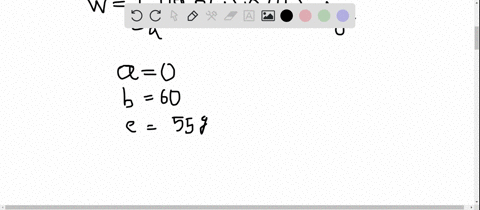Calculus for Scientists and Engineers: Early Transcendental

A 60 -m-long, 9.4 -mm-diameter rope hangs free from a ledge. The density of the rope is $55 \mathrm{g} / \mathrm{m} .$ How much work is needed to pull the entire rope to the ledge?

Applications of Integration
Physical Applications02:12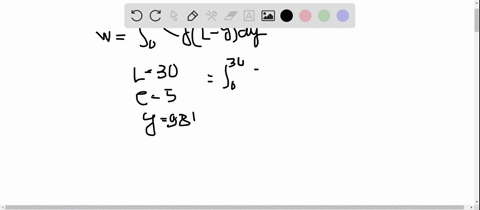Calculus for Scientists and Engineers: Early Transcendental

A 30 -m-long chain hangs vertically from a cylinder attached to a winch. Assume there is no friction in the system and that the chain has a density of $5 \mathrm{kg} / \mathrm{m}.$
a. How much work is required to wind the entire chain onto the cylinder using the winch?
b. How much work is required to wind the chain onto the cylinder if a $50-\mathrm{kg}$ block is attached to the end of the chain?

Applications of Integration
Physical Applications04:36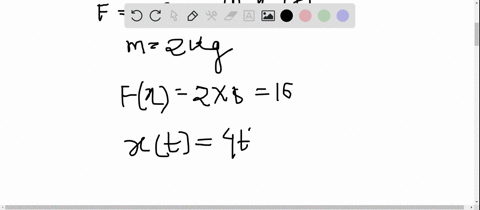Calculus for Scientists and Engineers: Early Transcendental

A rigid body with a mass of $2 \mathrm{kg}$ moves along a line due to a force that produces a position function $x(t)=4 t^{2},$ where $x$ is measured in meters and $t$ is measured in seconds. Find the work done during the first 5 s in two ways.
a. Note that $x^{\prime \prime}(t)=8 ;$ then use Newton's second law $\left(F=m a=m x^{\prime \prime}(t)\right)$ to evaluate the work integral $W=\int_{x_{0}}^{x_{f}} F(x) d x,$ where $x_{0}$ and $x_{f}$ are the initial and final positions, respectively.
b. Change variables in the work integral and integrate with respect to $t .$ Be sure your answer agrees with part (a).

Applications of Integration
Physical Applications06:48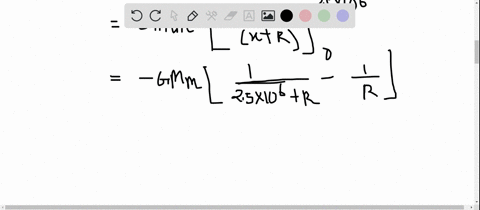Calculus for Scientists and Engineers: Early Transcendental

For large distances from the surface of Earth, the gravitational force is given by $F(x)=G M m /(x+R)^{2},$ where $G=6.7 \times 10^{-11} \mathrm{N} \cdot \mathrm{m}^{2} / \mathrm{kg}^{2}$ is the gravitational constant, $M=6 \times 10^{24} \mathrm{kg}$ is the mass of Earth, $m$ is the mass of the object in the gravitational field, $R=6.378 \times 10^{6} \mathrm{m}$ is the radius of Earth, and $x \geq 0$ is the distance above the surface of Earth (in meters).
a. How much work is required to launch a rocket with a mass of $500 \mathrm{kg}$ in a vertical flight path to a height of $2500 \mathrm{km}$ (from Earth's surface)?
b. Find the work required to launch the rocket to a height of $x$ kilometers, for $x>0.$
c. How much work is required to reach outer space $(x \rightarrow \infty) ?$
d. Equate the work in part (c) to the initial kinetic energy of the rocket, $\frac{1}{2} m v^{2},$ to compute the escape velocity of the rocket.

Applications of Integration
Physical Applications05:02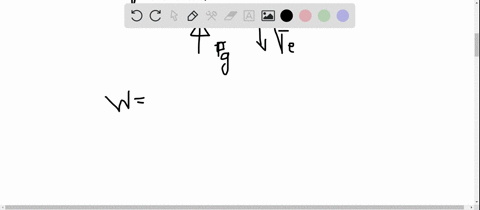Calculus for Scientists and Engineers: Early Transcendental

A $10-\mathrm{kg}$ mass is attached to a spring that hangs vertically and is stretched $2 \mathrm{m}$ from the equilibrium position of the spring. Assume a linear spring with $F(x)=k x.$
a. How much work is required to compress the spring and lift the mass $0.5 \mathrm{m} ?$
b. How much work is required to stretch the spring and lower the mass $0.5 \mathrm{m} ?$

Applications of Integration
Physical Applications02:58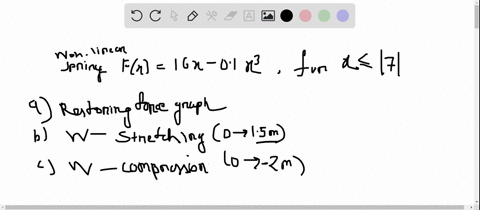Calculus for Scientists and Engineers: Early Transcendental

Hooke's law is applicable to idealized (linear) springs that are not stretched or compressed too far. Consider a nonlinear spring whose restoring force is given by $F(x)=16 x-0.1 x^{3},$ for $|x| \leq 7.$
a. Graph the restoring force and interpret it.
b. How much work is done in stretching the spring from its equilibrium position $(x=0)$ to $x=1.5 ?$
c. How much work is done in compressing the spring from its equilibrium position $(x=0)$ to $x=-2 ?$

Applications of Integration
Physical Applications1 2 3 4 5 ... 402